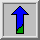# Rotate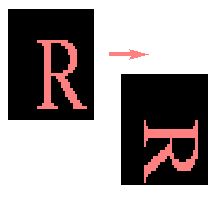Common Names: Rotation

## Brief Description

The rotation operator performs a geometric transform which maps the position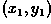of a picture element in an input image onto a position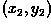in an output image by rotating it through a user-specified angle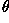about an origin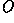. In most implementations, output locationswhich are outside the boundary of the image are ignored. Rotation is most commonly used to improve the visual appearance of an image, although it can be useful as a preprocessor in applications where directional operators are involved. Rotation is a special case of affine transformation.## How It Works

The rotation operator performs a transformation of the form: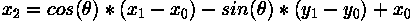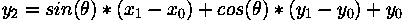where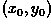are the coordinates of the center of rotation (in the input image) andis the angle of rotation with clockwise rotations having positive angles. (Note here that we are working in image coordinates, so the y axis goes downward. Similar rotation formula can be defined for when the y axis goes upward.) Even more than the translate operator, the rotation operation produces output locationswhich do not fit within the boundaries of the image (as defined by the dimensions of the original input image). In such cases, destination elements which have been mapped outside the image are ignored by most implementations. Pixel locations out of which an image has been rotated are usually filled in with black pixels.

The rotation algorithm, unlike that employed by translation, can produce coordinateswhich are not integers. In order to generate the intensity of the pixels at each integer position, different heuristics (or re-sampling techniques} may be employed. For example, two common methods include:

• Allow the intensity level at each integer pixel position to assume the value of the nearest non-integer neighbor.

• Calculate the intensity level at each integer pixel position based on a weighted average of the n nearest non-integer values. The weighting is proportional to the distance or pixel overlap of the nearby projections.

The latter method produces better results but increases the computation time of the algorithm.## Guidelines for Use

A rotation is defined by an angleand an origin of rotation. For example, consider the image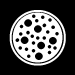whose subject is centered. We can rotate the image through 180 degrees about the image (and circle) center at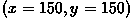to produce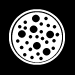If we use these same parameter settings but a new, smaller image, such as the 222×217 size artificial, black-on-white image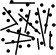we achieve poor results, as shown in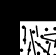because the specified axis of rotation is sufficiently displaced from the image center that much of the image is swept off the page. Likewise, rotating this image through avalue which is not an integer multiple of 90 degrees (e.g. in this caseequals 45 degrees) rotates part of the image off the visible output and leaves many empty pixel values, as seen in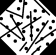(Here, non-integer pixel values were re-sampled using the first technique mentioned above.)

Like translation, rotation may be employed in the early stages of more sophisticated image processing operations. For example, there are numerous directional operators in image processing (e.g. many edge detection and morphological operators) and, in many implementations, these operations are only defined along a limited set of directions: 0, 45, 90, etc. A user may construct a hybrid operator which operates along any desired image orientation direction by first rotating an image through the desired direction, performing the edge detection (or erosion, dilation, etc.), and then rotating the image back to the original orientation. (See Figure 1.)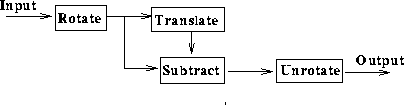Figure 1 A variable-direction edge detector.

As an example, consider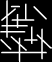whose edges were detected by the directional operator defined using translation giving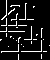We can perform edge detection along the opposite direction to that shown in the image by employing a 180 degree rotation in the edge detection algorithm. The result is shown in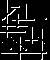Notice the slight degradation of this image due to rotation re-sampling.

## Interactive Experimentation

You can interactively experiment with this operator by clicking here.

## Exercises

1. Consider imagesandwhich contain L-shaped parts of different sizes. a) Rotate and translate one of the images such that the bottom left corner of the ``L'' is in the same position in both images. b) Using a combination of histograming, thresholding and pixel arithmetic (e.g. pixel subtraction) determine the approximate difference in size of the two parts.

2. Make a collage based on a series of rotations and pixel additions of imageYou should begin by centering the propeller in the middle of the image. Next, rotate the image through a series of 45 degree rotations and add each rotated version back onto the original. (Note: you can improve the visual appearance of the result if you scale the intensity values of each rotated propeller a few shades before adding it onto the collage.)

3. Investigate the effects of re-sampling when using rotation as a preprocessing tool in an image erosion application. First erode the imagesandusing a 90 degree directional erosion operator. Next, rotate the image through 90 degrees before applying the directional erosion operator along the 0 degree orientation. Compare the results.

## References

D. Ballard and C. Brown Computer Vision, Prentice-Hall, 1982, Appendix 1.

B. Horn Robot Vision, MIT Press, 1986, Chap. 3.

## Local Information